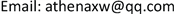1咸阳师范学院 资源环境与历史文化学院，陕西 咸阳

2中国地质调查局西安地质调查中心，陕西 西安

3西安向阳航天材料股份有限公司，陕西 西安1. 引言

2. 研究思路与数据来源

1) 研究思路

2) 数据来源

3. 实证研究

1) 研究区概况

2) 理论潜力的测算

Q t = S 0 − B t × P t = S 0 − B t × ( P 0 × ( 1 + r ) n ± Δ P ) (1)

3) 适宜性修正系数测算

f ( Y ) = ∑ i = 1 n ( S i S × n Y i ) (2)

4) 规划修正系数测算

f ( G ) = ( ∑ i = 1 n S G g = 1 i ) / S (3)

5) 测算农村建设用地整理实际潜力

Q = Q t × f ( Y ) × f ( G ) (4)

6) 农村建设用地整理潜力分级

Rural construction land rearrangement potential classification of Chengg

Ⅰ级[5.0, +∞)764.73611.5754.6
Ⅱ级[2.0, 5.0)534.4410.4336.64
Ⅲ级[0.01, 2.0)130.5698.158.76
Ⅳ级[0, 0.01)000

4. 结果分析

5. 结论与讨论